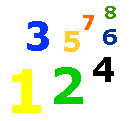# Division Facts

Division Facts Lesson & Worksheets - Dividing - Division Rules - My Schoolhouse - Online Learning

Basic division facts will help you solve any division problem.  You can use the multiplication facts you already know.  They will help you find the quotient of each division fact.

 6 X 2 = 12 therefore 12 -:- 2 = 6 or 5 X 3 = 15 therefore 15 -:- 5 = 3

Rules for dividing by 0.

Rule 1.

0 divided by any number (except 0) equals 0.

Examples:

0 -:- 3 = 0

0 -:- 9 = 0

0 -:- 5 = 0

Rule 2.

You cannot divide by 0.

Example: 3 -:- 0 is impossible to do.

Rules for dividing by 1.

Rule 1.

Any number (except 0) when divided by itself equals 1.

Examples:

3 -:- 3 = 1

7 -:- 7 = 1

9 -:- 9 = 1

Rule 2.

Any number divided by 1 equals that number.

Examples:

8 -:- 1 = 8

3 -:- 1 = 3

4 -:- 1 = 4

 2 as a divisor 3 as a divisor 4 as a divisor 5 as a divisor0 -:- 2 = 0 -:- 3 = 0 -:- 4 = 0 -:- 5 =2 -:- 2 = 3 -:- 3 = 4 -:- 4 = 5 -:- 5 =4 -:- 2 = 6 -:- 3 = 8 -:- 4 = 10 -:- 5 =6 -:- 2 = 9 -:- 3 = 12 -:- 4 = 15 -:- 5 =8 -:- 2 = 12 -:- 3 = 16 -:- 4 = 20 -:- 5 =10 -:- 2 = 15 -:- 3 = 20 -:- 4 = 25 -:- 5 =12 -:- 2 = 18 -:- 3 = 24 -:- 4 = 30 -:- 5 =14 -:- 2 = 21 -:- 3 = 28 -:- 4 = 35 -:- 5 =16 -:- 2 = 24 -:- 3 = 32 -:- 4 = 40 -:- 5 =18 -:- 2 = 27 -:- 3 = 36 -:- 4 = 45 -:- 5 =20 -:- 2 = 30 -:- 3 = 40 -:- 4 = 50 -:- 5 =

 Thank You for using My Schoolhouse

 Elementary & Middle School Lessons & Self-Correcting Worksheets for Children in all Subject Areas. Lessons & Worksheets in Math, Reading, Spelling, Science, Language, and Social Studies.     My Schoolhouse Copyright 1999-2021 by Educational Designers, LLC. All rights reserved. "This site uses cookies from Google to deliver its services and analyze traffic. Your IP address and user-agent are shared with Google along with performance and security metrics to ensure quality of service, generate usage statistics, and to detect and address abuse."